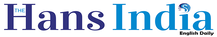Top

# Implied Volatilityx
Highlights

## In financial mathematics, the implied volatility of an option contract is that value of the volatility of the underlying instrument which, when input in an option pricing model (such as Black–Scholes) will return a theoretical value equal to the current market price of the option.

In financial mathematics, the implied volatility of an option contract is that value of the volatility of the underlying instrument which, when input in an option pricing model (such as Black–Scholes) will return a theoretical value equal to the current market price of the option.

A non-option financial instrument that has embedded optionality, such as an interest rate cap, can also have an implied volatility. Implied volatility, a forward-looking and subjective measure, differs from historical volatility because the latter is calculated from known past returns of a security.

An option pricing model, such as Black–Scholes, uses a variety of inputs to derive a theoretical value for an option. Inputs to pricing models vary depending on the type of option being priced and the pricing model used. However, in general, the value of an option depends on an estimate of the future realized price volatility of the underlying.

As stated by Brian Byrne, the implied volatility of an option is a more useful measure of the option's relative value than its price. The reason is that the price of an option depends most directly on the price of its underlying asset.

If an option is held as part of a delta neutral portfolio (that is, a portfolio that is hedged against small moves in the underlying price), then the next most important factor in determining the value of the option will be its implied volatility.

Implied volatility is so important that options are often quoted in terms of volatility rather than price, particularly between professional traders. Another way to look at implied volatility is to think of it as a price, not as a measure of future stock moves. In this view it simply is a more convenient way to communicate option prices than currency.

Volatility instruments are financial instruments that track the value of implied volatility of other derivative securities. For instance, the CBOE Volatility Index (VIX) is calculated from a weighted average of implied volatilities of various options on the S&P 500 Index.

There are also other commonly referenced volatility indices such as the VXN index (Nasdaq 100 index futures volatility measure), the QQV (QQQ volatility measure), IVX - Implied Volatility Index (an expected stock volatility over a future period for any of US securities and exchange traded instruments), as well as options and futures derivatives based directly on these volatility indices themselves.Next Story
More Stories